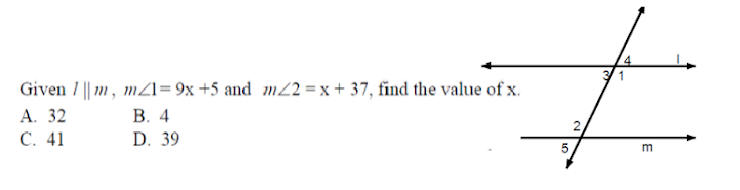Activity 1-5 On Line practice
Identify this picture *
1 point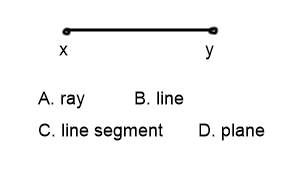Find <3 *
1 point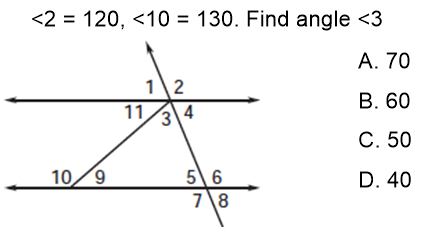Find distance *
1 point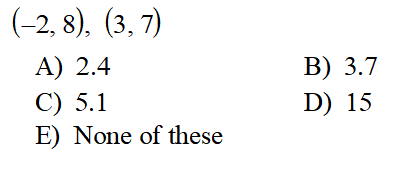*
1 point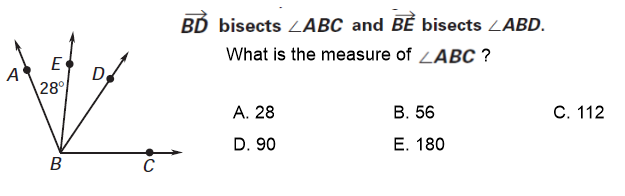Period *
F is between E and G. If EF = 5x + 15, FG = 53, and EG = 143. Find x. *
1 point
C is the midpoint of x and y. xy is 6x - 8 and xc is 2x + 1. Find x. *
1 point
What MUST BE TRUE about <3 and <5? *
1 point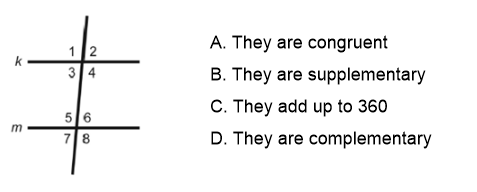Angle P and Q are complementary. If <P = 53, what is the measure of <Q? *
1 point
Last Name *
find angle x. *
1 point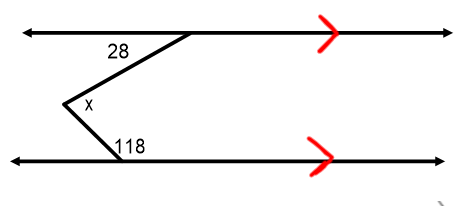Find x *
1 point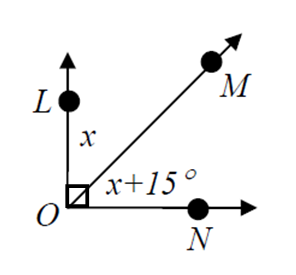Angle bisectors... make sure you read the directions. It doesn't ask for x. *
1 point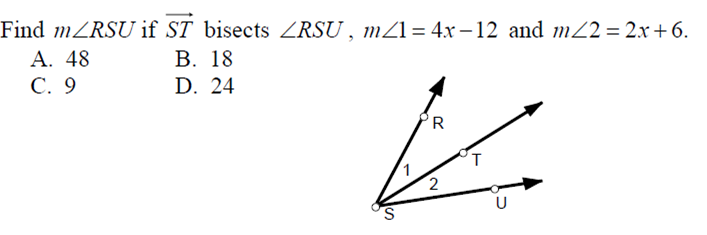Find angle x. *
1 point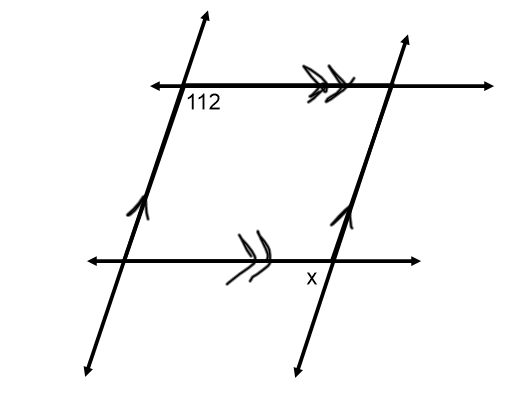Name the relationship *
1 point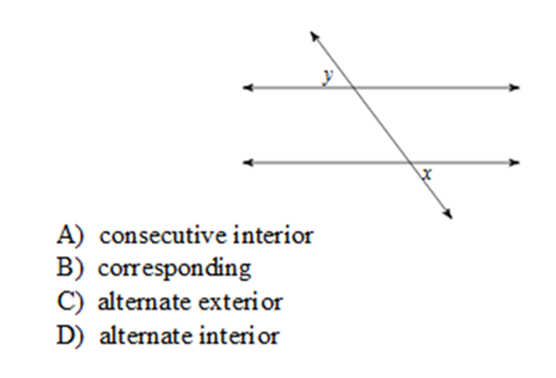Find y. You will need x first and sub it in. *
1 point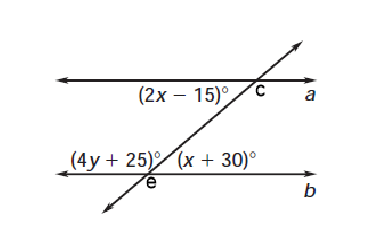Interior of angles *
1 point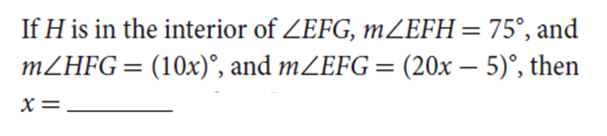Midpoint formula *
1 point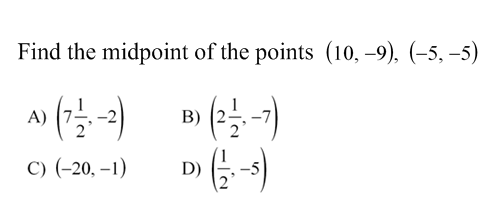2 angles are supplementary angle A is 3x + 12 and Angle B is 5x - 4. Find the measure of angle A. *
1 point
What is the measure of <1... You will need x. *
1 point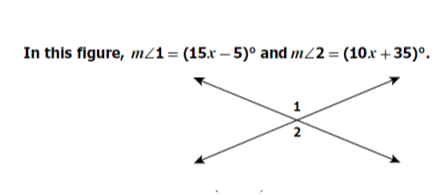Find the distance *
1 point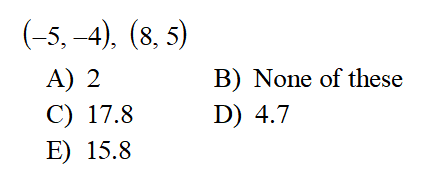parallel lines *
1 point